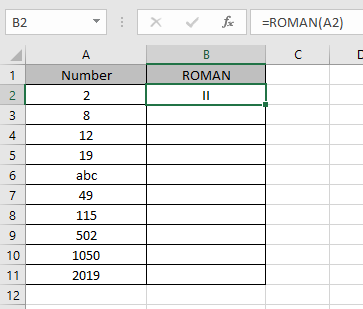# How to use the ROMAN Function in Excel

ROMAN function is a mathematical conversion operator used to convert the arabic numeral representation to ROMAN numeral as text. ROMAN numerals to arabic are shown below.

 ARABIC ROMAN 1 I 5 V 10 X 50 L 100 C 500 D 1000 M

The ROMAN function converts Arabic numeral to roman as text
Syntax:

=ROMAN (number, [form])

Number : Arabic numeral
[form] : [optional] a number specifying the type roman numeral you wish.
Let’s understand this function using it in an example.Here we have some values which we will be providing to the function and to see what the function returns.
Use the formula:

=ROMAN (A2)

A2 : arabic number which should be positive and less than 3999.
Here argument to the formula is taken as cell reference.As you can see the formula returns the ROMAN numeral as text from the input arabic numeral representation
Now copy the formula in other cells using Ctrl + D shortcut key.
These are the results returned by the ROMAN function.As you can see all the input arabic numerals gets converted to ROMAN as text.

Use ROMAN function with other function to get results.

Notes:

1. The function returns #VALUE! Error, if the argument to the function is negative numbers or text given in as cell reference.
2. If the number is in decimal then excel truncates it to integer and then return the value
3. The number should be positive and less than 3999.
4. The ROMAN function does the opposite of the ARABIC function in excel.
5. The form argument in the function returns the type of roman numeral you wish. There are few types of numeral you can select from:
 Form Type 0 Classic (default) 1 More concise style 2 More concise style 3 More concise style 4 Simplified style TRUE Classic style FALSE Simplified style

Hope you understood how to use ROMAN function and referring cell in Excel. Explore more articles on Excel conversion functions here. Please feel free to state your query or feedback for the above article.

Related Articles:

How to use the ARABIC Function in Excel

Popular Articles:

50 Excel Shortcuts to Increase Your Productivity

How to use the VLOOKUP Function in Excel

How to use the COUNTIF in Excel 2016

How to use the SUMIF Function in Excel

Terms and Conditions of use

The applications/code on this site are distributed as is and without warranties or liability. In no event shall the owner of the copyrights, or the authors of the applications/code be liable for any loss of profit, any problems or any damage resulting from the use or evaluation of the applications/code.